# Measure areas

The area of a parallelogram is measured in the same way as the area for a rectangle or a square. The height of a parallelogram is the distance between the two horizontal sides.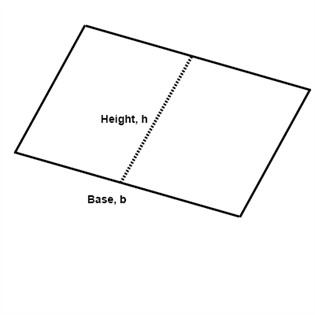$A=b\cdot h$

Example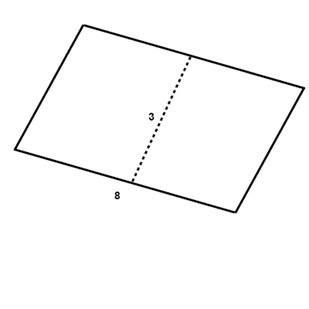$A=b\cdot h$

$A=8\cdot 3$

$A=24\: in^{2}$

As we showed in an earlier section we can divide a square or a quadrilateral into two triangles. This gives us that the area of a triangle is half the area of the quadrilateral with the same base and height.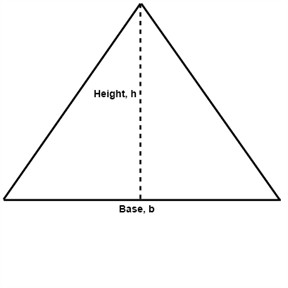If the area of a parallelogram is A=b ∙ h then the area for a triangle is A=0.5b ∙h or

$A=\frac{1}{2}b\cdot h$

Example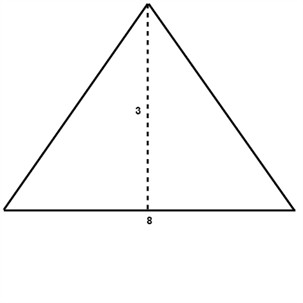$\\ A=\frac{1}{2}b\cdot h\\\\\\ \: \: \: A=\frac{1}{2}\cdot 8\cdot 3 \\\\\\ A=\frac{1}{2}\cdot 24\\\\\\ \, A=12\: in^{2} \\$

A trapezoid is a quadrilateral with two parallel sides. Both parallel sides are called bases.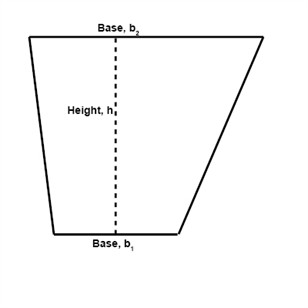To find the area of a trapezoid we need to divide it into two triangles. Both triangles produced have the height h.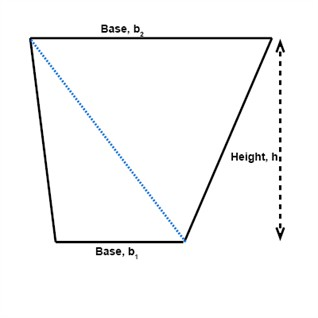The area of the trapezoid is the sum of the areas of the two triangles.

$\begin{matrix} A_{1}=\frac{1}{2}b_{1}\cdot h & \: \: \:and \: \: &A_{2}=\frac{1}{2} b_{2}\cdot h \end{matrix}$

$A=\frac{1}{2}b_{1}\cdot h+\frac{1}{2} b_{2}\cdot h$

$A=\frac{1}{2}h\cdot (b_{1}+b_{2})$

Example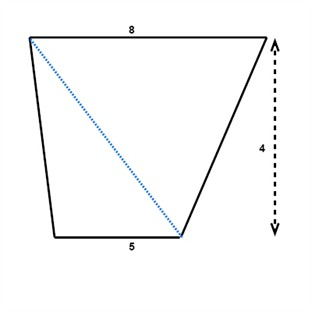$A=\frac{1}{2}\cdot 4\cdot (5+8)$

$A=2\cdot 13$

$A=26\, in^{2}$

The area of a circle is different from the areas of a triangle or a quadrilateral. The area formula of a circle is:

$A=\pi r^{2}$

We get the area of a circle by multiplying π by the radius, r, squared.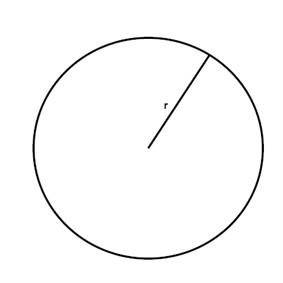Example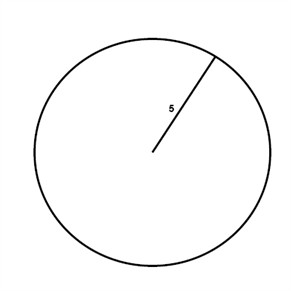$A=\pi r^{2}$

$A=\pi \cdot 5^{2}$

$A=\pi \cdot 25$

$A\approx 75.5\, in^{2}$

Find the areas